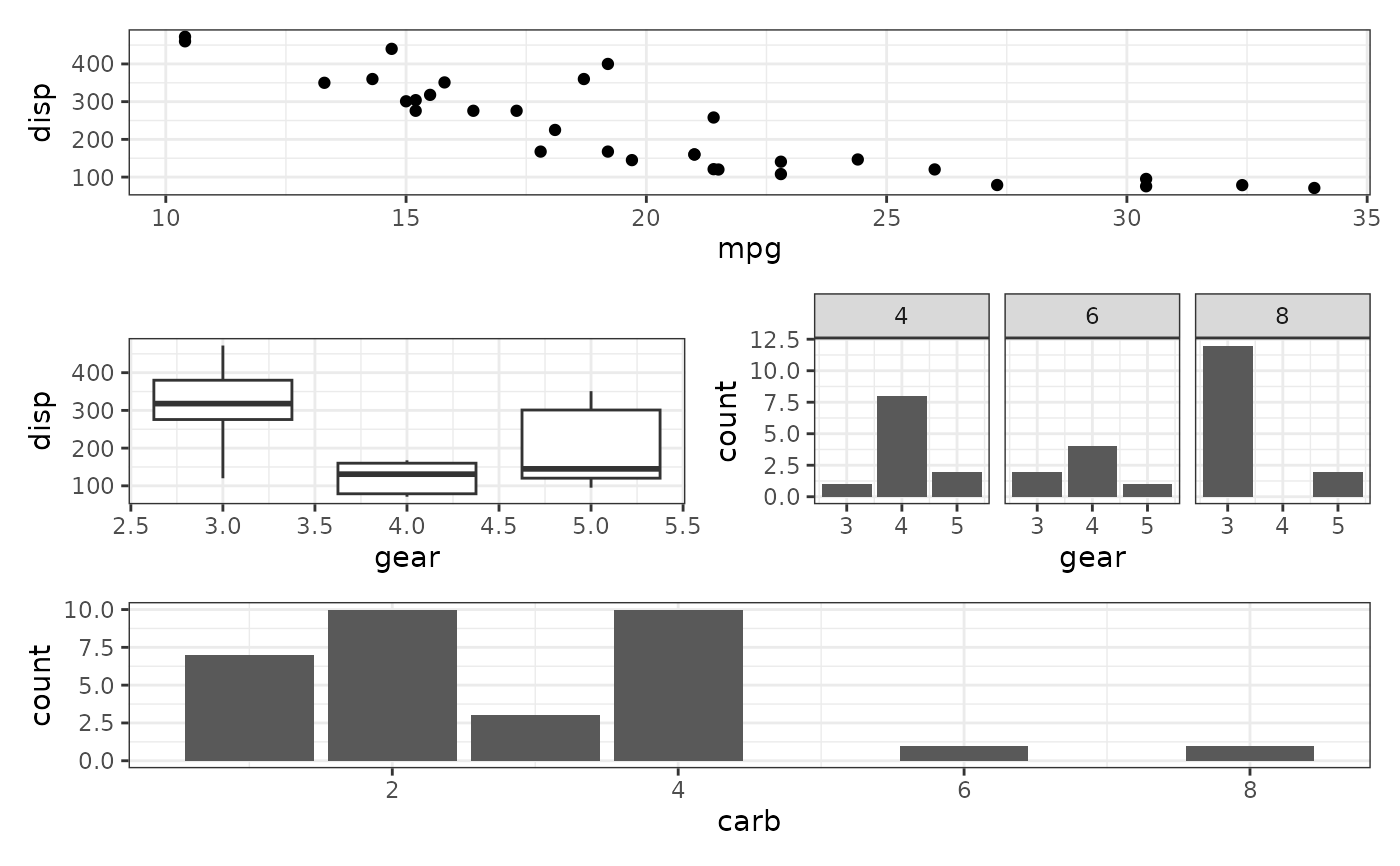In addition to the + operator known in ggplot2, patchwork defines logic for some of the other operators that aids in building up your plot composition and reduce code-reuse.

# S3 method for ggplot
-(e1, e2)

# S3 method for ggplot
/(e1, e2)

# S3 method for ggplot
|(e1, e2)

# S3 method for gg
*(e1, e2)

# S3 method for gg
&amp;(e1, e2)

Arguments

e1 A ggplot or patchwork object A ggplot or patchwork object in case of /, or a gg object such as a geom or theme specification in case of * and &

Value

A patchwork object

Details

patchwork augment the + operator from ggplot2 and allows the user to add full ggplot objects together in order to compose them into the same view. The last added plot is always the active one where new geoms etc. are added to. Another operator that is much like it, but not quite, is -. It also adds plots together but instead of adding the right hand side to the patchwork defined in the left hand side, it puts the left hand side besides the right hand side in a patchwork. This might sound confusing, but in essence - ensures that the right and left side are put in the same nesting level (+ puts the right side into the left side). Using - might seem unintuitive if you think of the operator as "subtract", but look at it as a hyphen instead (the underlying reason is that - is the only operator in the same precedence group as +).

Often you are interested in creating single column or single row layouts. patchwork provides | (besides) and / (over) operators to support stacking and packing of plots. See the exampels for their use.

In order to reduce code repetition patchwork provides two operators for adding ggplot elements (geoms, themes, facets, etc.) to multiple/all plots in a patchwork. * will add the element to all plots in the current nesting level, while & will recurse into nested patches.

Examples

library(ggplot2) p1 <- ggplot(mtcars) + geom_point(aes(mpg, disp)) p2 <- ggplot(mtcars) + geom_boxplot(aes(gear, disp, group = gear)) p3 <- ggplot(mtcars) + geom_bar(aes(gear)) + facet_wrap(~cyl) p4 <- ggplot(mtcars) + geom_bar(aes(carb)) # Standard addition vs division p1 + p2 + p3 + plot_layout(ncol = 1)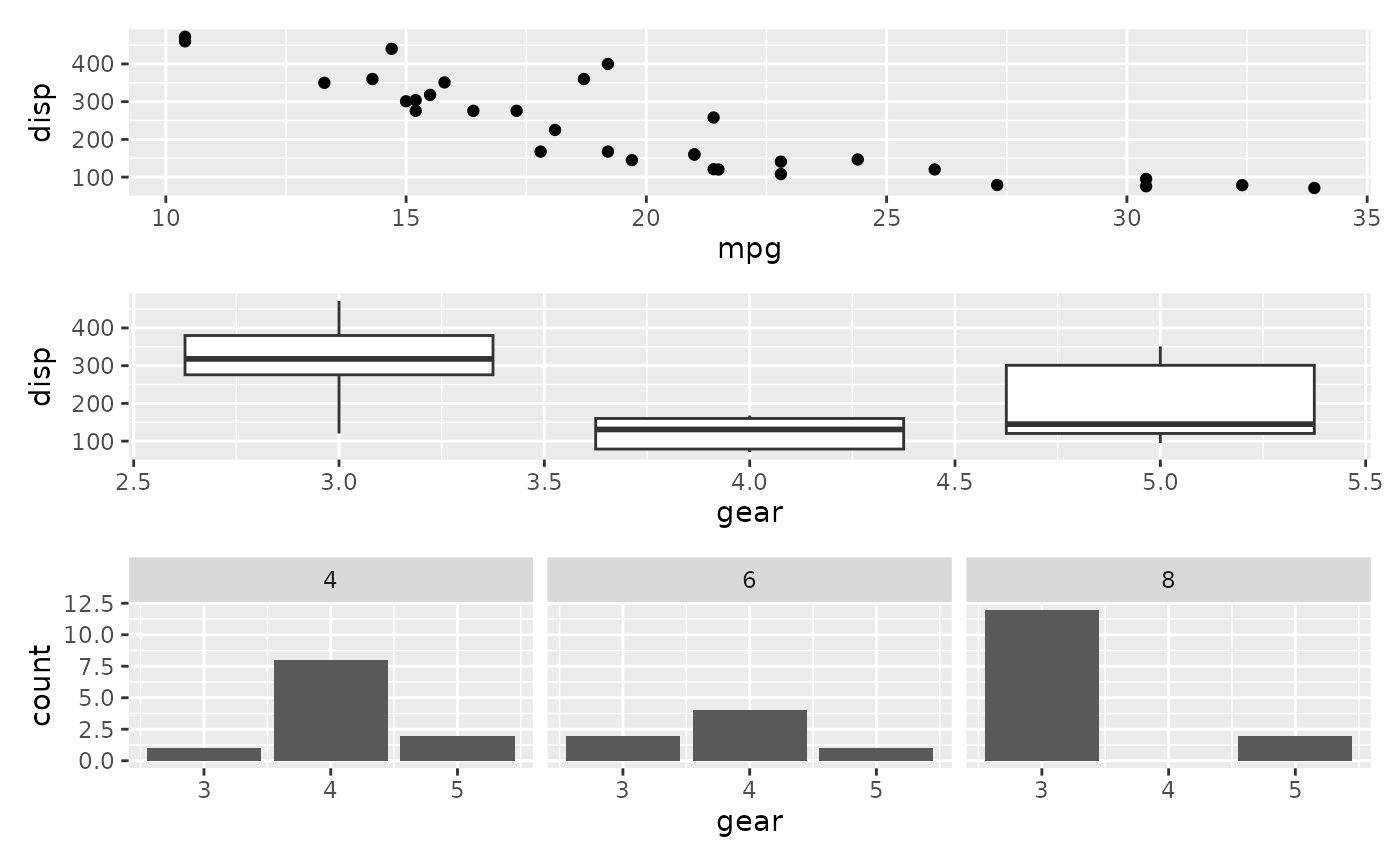p1 + p2 - p3 + plot_layout(ncol = 1)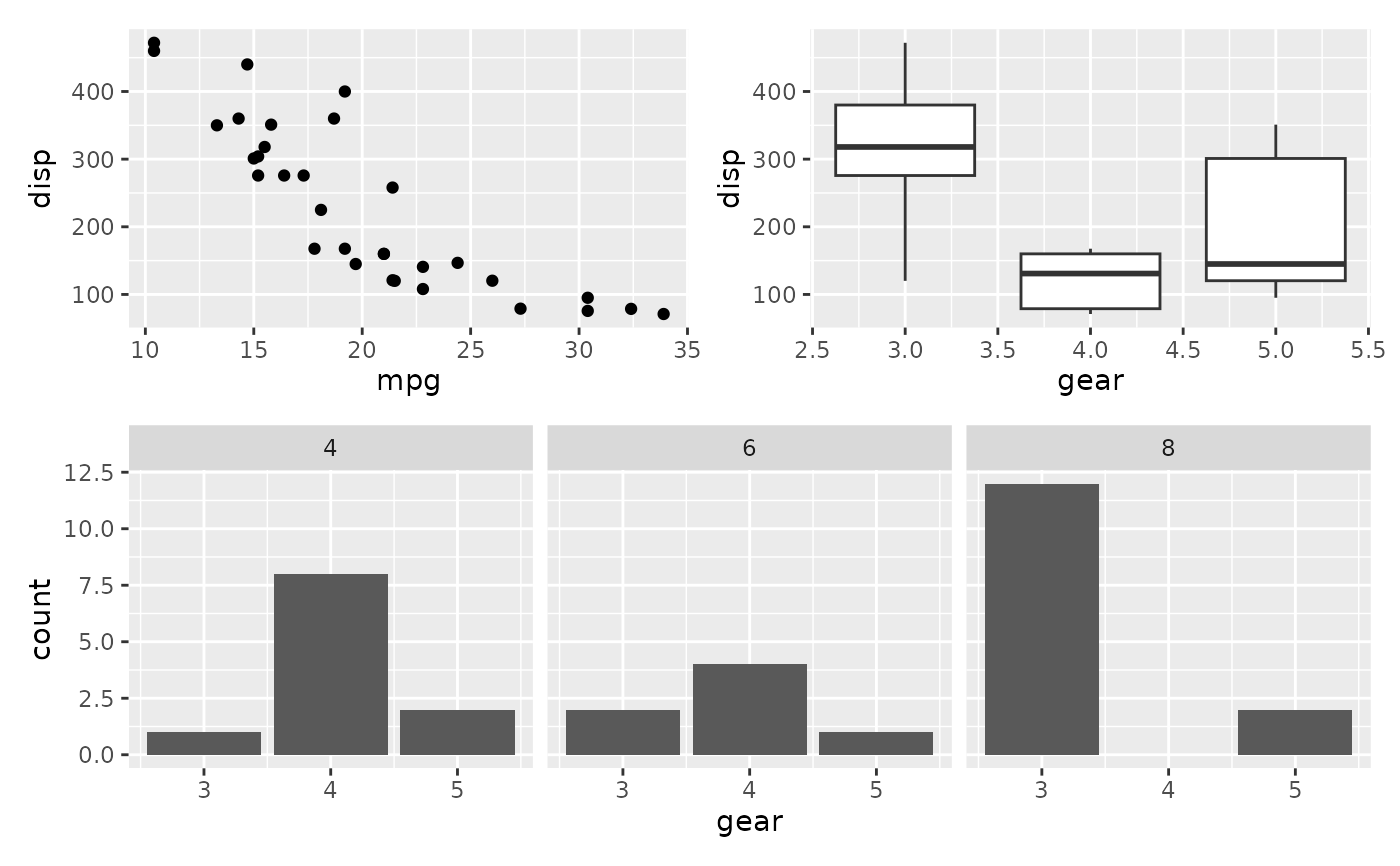# Stacking and packing (p1 | p2 | p3) / p4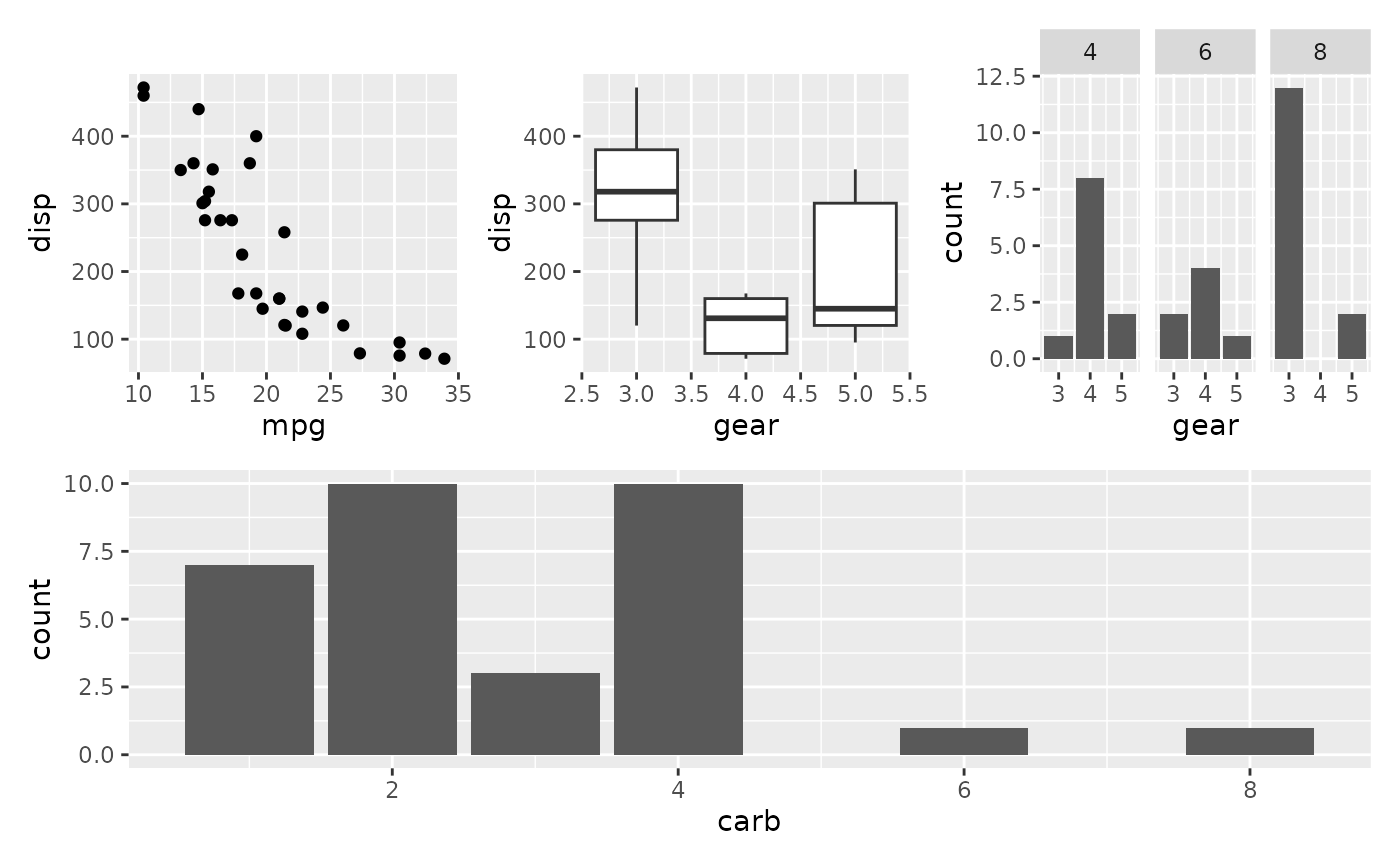# Add elements to the same nesting level (p1 + (p2 + p3) + p4 + plot_layout(ncol = 1)) * theme_bw()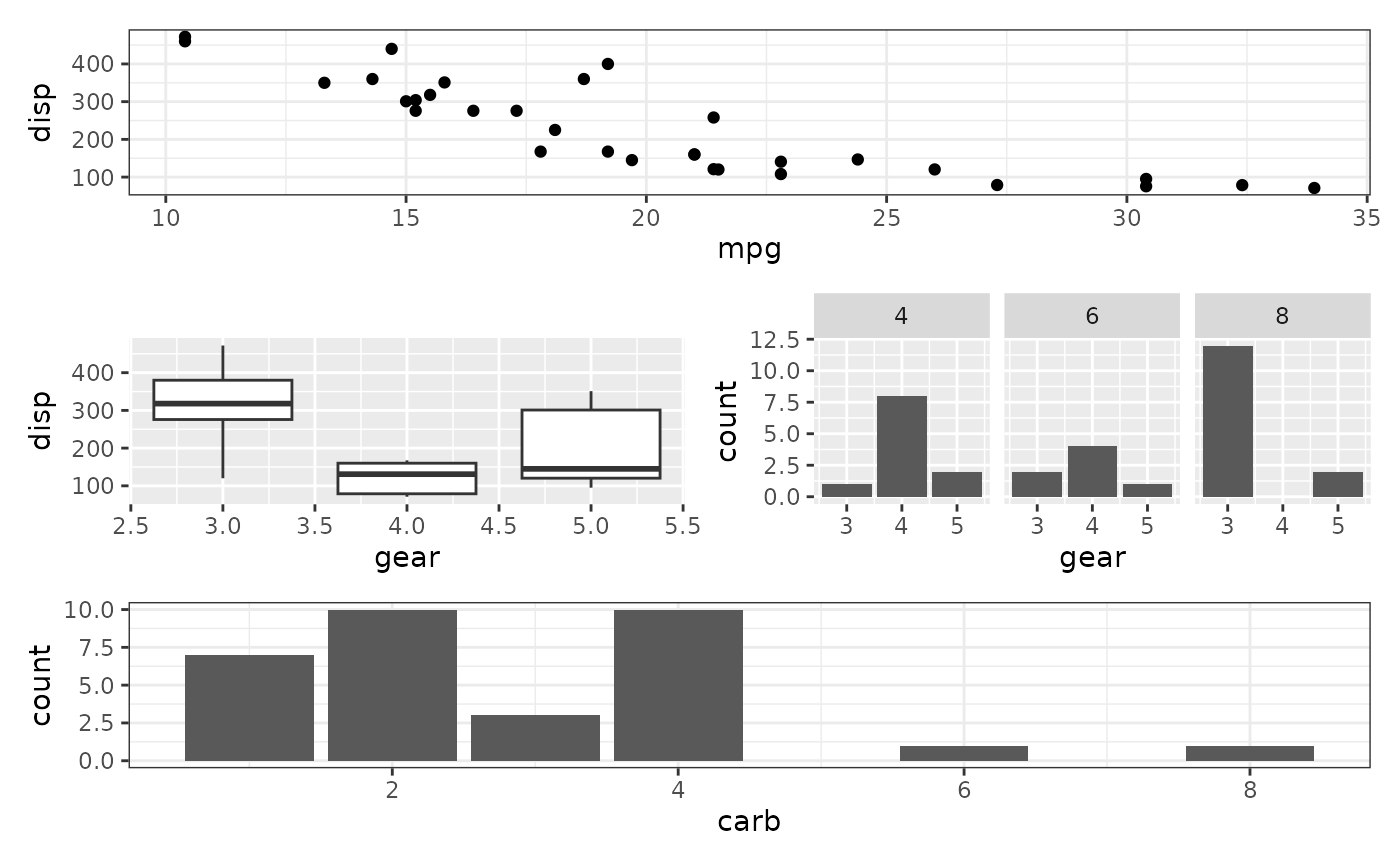# Recurse into nested plots as well (p1 + (p2 + p3) + p4 + plot_layout(ncol = 1)) & theme_bw()